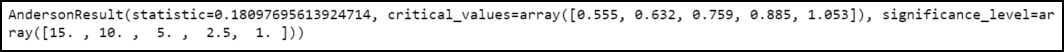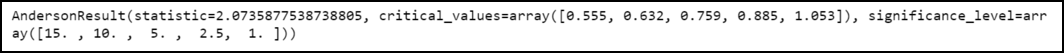GFG App
Open AppBrowser
Continue

# How to Perform an Anderson-Darling Test in Python

Anderson-Darling test: Its full name is Anderson-Darling Goodness of Fit Test (AD-Test) and it is used to measure the extent to which our data fits with the specified distribution. Mostly this test is used to find whether the data follows a normal distribution.

Syntax to install scipy and numpy library:

`pip3 install scipy numpy`

Scipy is a python library used for scientific computation. It provides anderson() function to conduct the Anderson-Darling test.

anderson() function:

Syntax:

anderson(arr, dist=’norm’)

Parameters:

arr: It is an array of sample data

dist: It specifies the type of distribution to test against. By Default it is set to ‘norm’ but we can also use ‘expon’ or ‘logistic.’

Example 1:

## Python3

 `# Python program to conduct Anderson-Darling Test ` ` `  `# Importing libraries ` `import` `numpy as np ` `from` `scipy.stats ``import` `anderson ` ` `  `# Creating data ` `np.random.seed(``0``) ` `data ``=` `np.random.normal(size``=``100``) ` ` `  `# Conduct Anderson-Darling Test  ` `anderson(data)`

Output:Output

The test statistic comes out to be 0.18. This value can be compared with each critical value that corresponds to each significance level to check whether the test outcomes are significant.

For example:

• The critical value for α = 0.01 is equal to 1.021. Because this test statistic (0.18) is not greater than this critical value hence the test result cannot be said as significant at a significance level of 0.01.
• The critical value for α = 0.025 is equal to 0.858. Because this test statistic (0.18) is not greater than this critical value hence the test result cannot be said as significant at a significance level of 0.025.

Since the test results are not significant at any significance level that implies that we cannot reject the null hypothesis of this test. Therefore, we don’t have sufficient proof to claim that the given data is not normally distributed.

Example 2:

Now let us consider conducting the Anderson-Darling Test on a sample of 100 random integers between 0 and 20.

## Python3

 `# Python program to conduct Anderson-Darling Test ` ` `  `# Importing libraries ` `import` `numpy as np ` `from` `scipy.stats ``import` `anderson ` ` `  `# Creating data ` `np.random.seed(``0``) ` `data ``=` `np.random.randint(``0``, ``20``, size``=``100``) ` ` `  `# Conduct Anderson-Darling Test ` `anderson(data) `

Output:Output

The test statistic comes out to be 2.073. This value can be compared with each critical value that corresponds to each significance level to check whether the test outcomes are significant.

For example:

• The critical value for α = 0.01 is equal to 1.021. Because this test statistic (0.18) is not greater than this critical value hence the test result cannot be said as significant at a significance level of 0.01.
• The critical value for α = 0.025 is equal to 0.858. Because this test statistic (0.18) is not greater than this critical value hence the test result cannot be said as significant at a significance level of 0.025.

Since the test results are not significant at any significance level that implies that we cannot reject the null hypothesis of this test. Therefore, we don’t have sufficient proof to claim that the given data is not normally distributed.

My Personal Notes arrow_drop_up